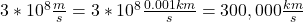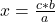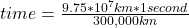## A space probe on the surface of Mars sends a radio signal back to the Earth, a distance of 9.75 ✕ 107 km. Radio waves travel at the speed of

Question

A space probe on the surface of Mars sends a radio signal back to the Earth, a distance of 9.75 ✕ 107 km. Radio waves travel at the speed of light (3.00 ✕ 108 m/s). How many seconds does it take for the signal to reach the Earth?

in progress 0
2 weeks 2021-09-02T06:02:10+00:00 1 Answers 0 views 0

It takes 325 seconds for the signal to reach Earth.

Explanation:

First, you must make a unit change from m/s to km/s in order to make a comparison with the distance of the radio signal sent to Earth. For that, you know that 1 m is 0.001 km. So:The rule of three or is a way of solving problems of proportionality between three known values and an unknown value, establishing a relationship of proportionality between all of them. That is, what is intended with it is to find the fourth term of a proportion knowing the other three. Remember that proportionality is a constant relationship or ratio between different magnitudes.

If the relationship between the magnitudes is direct, that is, when one magnitude increases, so does the other (or when one magnitude decreases, so does the other) , the direct rule of three must be applied. To solve a direct rule of three, the following formula must be followed:

a ⇒ b

c ⇒ xIn this case, the rule of three is applied as follows: if by definition of speed, 300,000 km of light are traveled in 1 second, 9.75 * 10⁷ km in how long are they traveled?time=325 seconds

It takes 325 seconds for the signal to reach Earth.